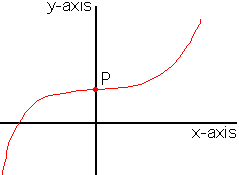How do you use and find the y-intercept? Kayla Boylan, 7th grade student Hi Kalya, The y-intercept of a graph is the y-coordinate of the point where the graph crosses the y-axis.In the diagram the point where the graph crosses the y-axis is P. Since P is on the y-axis its coordinates are (0,b) for some number b. b is the y-intercept. To find the y-intercept substitute x = 0 in the equation and solve for y. For example if you have the graph of y = 6 x2 + 2 x + 8 then substitute x = 0 to get y = 0 + 0 + 8 = 8 and thus y = 8 is the y-intercept. Cheers,Penny

Go to Math Central